Download Presentation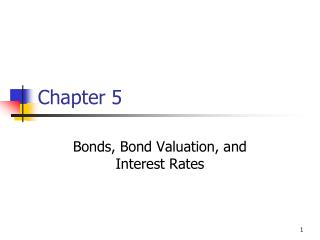Chapter 5

# Chapter 5 - PowerPoint PPT Presentation

Chapter 5. Bonds, Bond Valuation, and Interest Rates. Topics in Chapter. Key features of bonds Bond valuation Measuring yield Assessing risk. What is a Bond?. Key Features of a Bond. Par value: Face amount; paid at maturity. Assume \$1,000.I am the owner, or an agent authorized to act on behalf of the owner, of the copyrighted work described.
Download Presentation## Chapter 5

An Image/Link below is provided (as is) to download presentation

Download Policy: Content on the Website is provided to you AS IS for your information and personal use and may not be sold / licensed / shared on other websites without getting consent from its author.While downloading, if for some reason you are not able to download a presentation, the publisher may have deleted the file from their server.

- - - - - - - - - - - - - - - - - - - - - - - - - - E N D - - - - - - - - - - - - - - - - - - - - - - - - - -
Presentation Transcript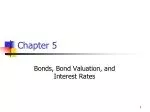### Chapter 5

Bonds, Bond Valuation, and Interest RatesTopics in Chapter
• Key features of bonds
• Bond valuation
• Measuring yield
• Assessing risk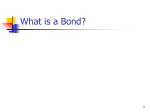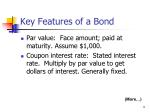Key Features of a Bond
• Par value: Face amount; paid at maturity. Assume \$1,000.
• Coupon interest rate: Stated interest rate. Multiply by par value to get dollars of interest. Generally fixed.

(More…)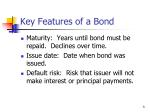Key Features of a Bond
• Maturity: Years until bond must be repaid. Declines over time.
• Issue date: Date when bond was issued.
• Default risk: Risk that issuer will not make interest or principal payments.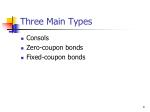Three Main Types
• Consols
• Zero-coupon bonds
• Fixed-coupon bonds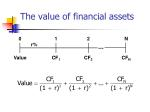0

1

2

N

r%

...

Value

CF1

CF2

CFN

The value of financial assets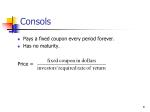Consols
• Pays a fixed coupon every period forever.
• Has no maturity.

Price =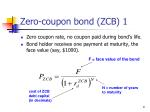Zero-coupon bond (ZCB) 1
• Zero coupon rate, no coupon paid during bond’s life.
• Bond holder receives one payment at maturity, the face value (say, \$1000).

F = face value of the bond

N = number of years to maturity

cost of ZCB debt capital (in decimals)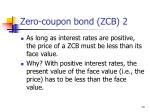Zero-coupon bond (ZCB) 2
• As long as interest rates are positive, the price of a ZCB must be less than its face value.
• Why? With positive interest rates, the present value of the face value (i.e., the price) has to be less than the face value.ZCB Problems

1) Find the price of a ZCB with 20 years to maturity, par value of \$1000 and a required rate of return of 15% p.a.

N=20, I/Y=15, FV=1000, PMT=0. Price = \$61.10

2) XYZ Corp.’s ZCB has a market price of \$ 354. The bond has 16 years to maturity and its face value is \$1000. What is the cost of debt for the ZCB (i.e., the required rate of return).

PV=-354, FV=1000, N=16, PMT=0.

Required rate of return/ Cost of debt =6.71%Fixed-coupon bond (FCB) 1
• Firm pays a fixed amount (‘coupon’) to the investor every period until bond matures.
• At maturity, firm pays face value of the bond to investor.
• Face value is also called par value. Unless otherwise stated, always assume face value to be \$1000.
• Period: can be year, half-year (6 months), quarter (3 months).Fixed-coupon bond (FCB) 2
• FCB gives you a stream of fixed payments plus a single payment (face value) at maturity.
• This cash flow stream is just an annuity plus a single cash flow at maturity.
• Therefore, we calculate the price of a FCB by finding the PV of the annuity and the single payment.Fixed-coupon bond (FCB) 3

Price of the FCB, PFCB

Fixed periodic coupon

Number of periods to maturity

Face value

Cost of debt capital0

1

2

10

Value of a 10-year, 10% coupon bond if rd = 10%

10%

...

V = ?

100

100

100 + 1,000

\$100

\$100

\$1,000

V

=

.

.

.

+

+

+

B

(1 + rd)1

(1 + rd)N

(1 + rd)N

= \$90.91 + . . . + \$38.55 + \$385.54

= \$1,000.PV annuity

PV maturity value

Value of bond

\$ 614.46

385.54

\$1,000.00

=

=

=

INPUTS

10 10 100 1000

N I/YR PV PMT FV

-1,000

OUTPUT

The bond consists of a 10-year, 10% annuity of \$100/year plus a \$1,000 lump sum at t = 10:INPUTS

10 13 100 1000

N I/YR PV PMT FV

-837.21

OUTPUT

What would happen if r = 13%?

If coupon rate < rd, then price < par, sells at a discount.INPUTS

10 7 100 1000

N I/YR PV PMT FV

-1,210.71

OUTPUT

What would happen if r= 7%?

If coupon rate > rd, then price > par, sells at a premium.Coupon rate vs. rd, and price
• If coupon rate < rd, bond sells at a discount.
• If coupon rate = rd, bond sells at its par value.
• If coupon rate > rd, bond sells at a premium.
• If rd rises, price falls.
• Price = par at maturity.Example 1
• A 10-year annual coupon bond was issued four years ago at par. Since then the bond’s yield to maturity (YTM) has decreased from 9% to 7%. Which of the following statements is true about the current market price of the bond?
• The bond is selling at a discount
• The bond is selling at par
• The bond is selling at a premium
• The bond is selling at book value
• Insufficient informationExample 2
• One year ago Pell Inc. sold 20-year, \$1,000 par value, annual coupon bonds at a price of \$931.54 per bond. At that time the market rate (i.e., yield to maturity) was 9 percent. Today the market rate is 9.5 percent; therefore the bonds are currently selling:
• at a discount.
• at a premium.
• at par.
• above the market price.
• not enough information.Bond Values over Time (1)
• Suppose a 10% fixed coupon bond was issued 20 years ago and now has 10 years to maturity. What would happen to its value over time if the required rate of return remained at 10%, or at 13%, or at 7%?
• See next slide.Bond Value over Time (2)

rd = 7%.

1,372

1,211

rd = 10%.

M

1,000

837

rd= 13%.

775

30 25 20 15 10 5 0Bond Value over Time (3)
• At maturity, the value of any bond must equal its par value.
• The value of a premium bond would decrease to \$1,000.
• The value of a discount bond would increase to \$1,000.
• A par bond stays at \$1,000 if rd remains constant.What’s “yield to maturity”?
• YTM is the rate of return earned on a bond held to maturity. It assumes the bond will not default.Definitions

Annual coupon pmt

Current price

Current yield =

Capital gains yield =

= YTM = +

Change in price

Beginning price

Exp total

return

Exp

Curryld

Exp cap

gains yld\$90

\$887

Current yield =

= 0.101466 = 10.1466%.

9% coupon, 10-year bond, P = \$887, and YTM = 10.9117%YTM = Current yield + Capital gains yield.

Cap gains yield = YTM - Current yield

= 10.9117% - 10.1466%

= 0.7651%.

Could also find p1,and then (p1-p0)/p0, same answer. How to find p1?INPUTS

2N rd/2 OK INT/2 OK

N I/YR PV PMT FV

OUTPUT

Semiannual Bonds

1. Multiply years by 2 to get periods = 2N.

2. Divide nominal rate by 2 to get periodic rate = rd/2.

3. Divide annual INT by 2 to get PMT = INT/2.2(10) 13/2 100/2

20 6.5 50 1000

N I/YR PV PMT FV

-834.72

INPUTS

OUTPUT

Value of 10-year, 10% coupon, semiannual bond if rd = 13%.Find YTM, Coupon rate

1)A \$1,000 par value bond sells for \$863.05. It matures in 20 years, has a 10 percent coupon rate, and pays interest semi-annually. What is the bond’s yield to maturity on a per annum basis (to 2 decimal places)?

Verify that YTM = 11.80%

2) ABC Inc. just issued a twenty-year semi-annual coupon bond at a price of \$787.39. The face value of the bond is \$1,000, and the market interest rate is 9%. What is the annual coupon rate (in percent, to 2 decimal places)?

Verify that annual coupon rate = 6.69%Long FCB question
• HMV Inc. needs to raise funds for an expansion project. The company can choose to issue either zero-coupon bonds or semi-annual coupon bonds. In either case the bonds would have the SAME nominal required rate of return, a 20-year maturity and a par value of \$1,000. If the company issues the zero-coupon bonds, they would sell for \$153.81. If it issues the semi-annual coupon bonds, they would sell for \$756.32. What annual coupon rate is HMV Inc. planning to offer on the coupon bonds? State your answer in percentage terms, rounded to 2 decimal places.

Verify that annual coupon rate = 7.01%Callable Bonds and Yield to Call
• A 10-year, 10% semiannual coupon,\$1,000 par value bond is selling for\$1,135.90 with an 8% yield to maturity.It can be called after 5 years at \$1,050. How much is the yield to call?INPUTS

10 -1135.9 50 1050

N I/YR PV PMT FV

3.765 x 2 = 7.53%

OUTPUT

Nominal Yield to Call (YTC)Interest rate risk: Rising rd causes bond’s price to fall.

10-year

rd

Change

Change

1-year

5%

\$1,048

\$1,386

38.6%

4.8%

10%

1,000

1,000

25.1%

4.4%

15%

956

749Interest rate risk and time to maturity
• The 10-year bond is more sensitive to interest rate changes because of longer time to maturity, and hence has higher interest rate risk.Term Structure Yield Curve
• Term structure of interest rates: the relationship between interest rates (or yields) and maturities.
• A graph of the term structure is called the yield curve.BB-Rated

Yield Curve

Interest

Rate (%)

15

10

5

Years to

Maturity

0

0

1

5

10

15

20IP, DRP, LP, Bond Spreads
• Inflation Premium (IP)
• Default Risk Premium (DRP)
• Liquidity Premium (LP)

Bond’s of large companies often have very small LPs. Bond’s of small companies often have LPs as high as 2%.

• Bond Spread: the difference between a corporate bond’s yield and a Treasury security’s yield of the same maturity.Bankruptcy 1
• Two main chapters of Federal Bankruptcy Act:
• Chapter 11, Reorganization
• Chapter 7, Liquidation
• Typically, the management wants Chapter 11, creditors may prefer Chapter 7.Bankruptcy 2
• If a company can’t meet its obligations, it files under Chapter 11. That stops creditors from foreclosing, taking assets, and shutting down the business.
• Company has 120 days to file a reorganization plan.
• Court appoints a “trustee” to supervise reorganization.
• Management usually stays in control.Bankruptcy 3
• Company must demonstrate in its reorganization plan that it is “worth more alive than dead.”
• Otherwise, judge will order liquidation under Chapter 7.If the company is liquidated, here’s the payment priority:
• Past due property taxes
• Secured creditors from sales of secured assets.
• Trustee’s costs
• Expenses incurred after bankruptcy filing
• Wages and unpaid benefit contributions, subject to limits
• Unsecured customer deposits, subject to limits
• Taxes
• Unfunded pension liabilities
• Unsecured creditors
• Preferred stock
• Common stockAfter Chapter Homework
• Problems: 1, 2, 3, 7, 8, 9, 10, 11, 12, 13, 14, 16, 21